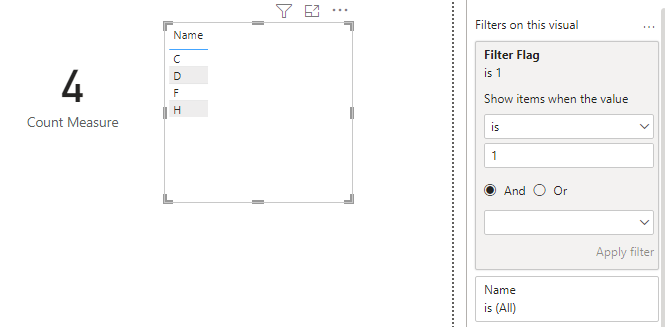cancel
Showing results for
Did you mean:Helper III

## Count/export empty and duplicate value

Hi,

I have the following table:

 Name Serial numer A 2DC25F B F3DGG C D E 5GERG F 6XDBD G 89DBBE H 6XDBD

The result would be 4  here. I would like to display the result in a card and can export these names.

1 ACCEPTED SOLUTIONSuper User

You can create a measure like

``````Duplicates and blanks =
VAR SummaryTable =
SUMMARIZE( 'Table', 'Table'[Serial numer] ),
"@num rows", CALCULATE( COUNTROWS( 'Table' ) )
)
RETURN
SUMX(
FILTER(
SummaryTable,
[@num rows] > 1 || ISBLANK( 'Table'[Serial numer] )
),
[@num rows]
)``````
3 REPLIES 3Community Support

Hi @vnqt

You can use this measure to count

``Count Measure = SUMX(FILTER(SUMMARIZE('Table','Table'[Serial numer],"Count",COUNT('Table'[Name])),[Count]>1),[Count])``

If you want to display the names in the report, you can use another measure as a filter. Add Name column to a table visual in the report, then add below measure to filter pane as a visual-level filter on this table visual. Set it to show items when value is 1.

``````Filter Flag =
var vSNs = SELECTCOLUMNS(FILTER(SUMMARIZE(ALL('Table'),'Table'[Serial numer],"Count",COUNT('Table'[Name])),[Count]>1),"SN",[Serial numer])
return
IF(SELECTEDVALUE('Table'[Serial numer]) IN vSNs, 1, 0)``````Best Regards,
Community Support Team _ Jing
If this post helps, please Accept it as Solution to help other members find it.Super User

You can create a measure like

``````Duplicates and blanks =
VAR SummaryTable =
SUMMARIZE( 'Table', 'Table'[Serial numer] ),
"@num rows", CALCULATE( COUNTROWS( 'Table' ) )
)
RETURN
SUMX(
FILTER(
SummaryTable,
[@num rows] > 1 || ISBLANK( 'Table'[Serial numer] )
),
[@num rows]
)``````Helper III